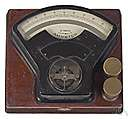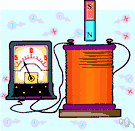# ampere

(redirected from Microamp)
Also found in: Thesaurus, Medical, Encyclopedia.

## am·pere

(ăm′pîr′)
n. Abbr. A
The basic unit of electric current, equal to one coulomb per second and equivalent to the current, flowing in two straight parallel wires of negligible cross section separated by a distance of one meter, that produces a force between the wires of 2.0 × 10-7 newtons per meter of length. The value of an ampere in the International System differs very slightly from that in the meter-kilogram-second-ampere system of units. See Table at measurement.

[After André Marie Ampère.]

## Ampère

(ˈæmpɛə; French ɑ̃pɛr)
n
(Biography) André Marie (ɑ̃dre mari). 1775–1836, French physicist and mathematician, who made major discoveries in the fields of magnetism and electricity

## ampere

(ˈæmpɛə)
n
1. (Units) the basic SI unit of electric current; the constant current that, when maintained in two parallel conductors of infinite length and negligible cross section placed 1 metre apart in free space, produces a force of 2 × 10–7 newton per metre between them. 1 ampere is equivalent to 1 coulomb per second
2. (Units) a former unit of electric current (international ampere); the current that, when passed through a solution of silver nitrate, deposits silver at the rate of 0.001118 gram per second. 1 international ampere equals 0.999835 ampere
Abbreviation: amp Symbol: A
[C19: named after André Marie Ampère]

## am•pere

(ˈæm pɪər, æmˈpɪər)

n.
the SI unit of electrical current, equal to a constant current that would produce a force of 2 x 10−7 newton per meter of length when maintained in two straight parallel conductors of infinite length and negligible circular cross section and placed one meter apart in a vacuum. Abbr.: A, amp.
[1881; after A. M. Ampère]

## Am•père

(ˈæm pɪər, æmˈpɪər, Fr. ɑ̃ˈpɛr)

n.
André Marie, 1775–1836, French physicist.

## am·pere

(ăm′pîr′)
A unit used to measure electric current. Electric current is measured by how great a charge passes a given point in a second. One ampere is equal to a flow of one coulomb per second.

## ampere

1. The unit of electric current in the international system.
2. (A) The unit for measuring electric current.
ThesaurusAntonymsRelated WordsSynonymsLegend:
 Noun 1ampere - a former unit of electric current (slightly smaller than the SI ampere)international amperecurrent unit - a measure of the amount of electric charge flowing past a circuit point at a specific time 2ampere - the basic unit of electric current adopted under the Systeme International d'Unites; "a typical household circuit carries 15 to 50 amps"amp, Acurrent unit - a measure of the amount of electric charge flowing past a circuit point at a specific timemilliampere, mA - one thousandth of an ampereabamp, abampere - a unit of current equal to 10 amperes
Translations
ampér
ampere
amper
ampeeri
amper
amper
amper
アンペア
amper
ampér
amper
ampere

## ampere

ampère [ˈæmpɛəʳ]
A. N
B. CPD ampere-hour Namperio-hora m

## ampere

ampère [ˈæmpɛər] (British) n

## ampère

(ˈӕmpeə) noun
(also amp (ӕmp) ) (often abbreviated to A when written) the unit by which an electric current is measured.
References in periodicals archive ?
Reactions were performed in 96-well optical plates (0.1 [micro]l MicroAmp, Applied Biosystems) and submitted to the following temperatures and cycles: a first cycle of 10 min denaturation at 95[degrees]C followed by 40 cycles of 15 s at 95[degrees]C and 1 min extension at 60[degrees]C.
Qualitative TSA used 10 [micro]M of hPPAR[beta]/[delta] LBD, 5x SYPRO[R] Orange (Sigma Aldrich) in buffer containing 20 mM HEPES pH 7.5, 200 mM NaCl, and 5% glycerol, and 30 [micro]M (~0.01 [micro]g/mL) of commercial agonists (1 protein: 3 ligand) or 140 [micro]g/mL of extracts from the tested library were added in a Microamp 96-well plate (Applied Biosystems).
These methods require a minimum number of dark I-V points (100 in our case) measured very accurately in the microamp range.
"So, for example, if the quiescent power is a microamp, then even if the load pulls only a nanoamp, ifs still going to consume a microamp of current.
This means the average current consumption of these devices is usually in the microamp range, so that even the smallest solar panel can easily keep these devices' small supercaps topped off.
Many instruments cannot make these low-level current measurements in the microamp range, and some cannot in the milliamp range.
Twenty-five microliters of the PCR component mix was added to each well of the custom array, and the plate was sealed with MicroAmp Optical Adhesive Film.
Microamp supply current has been the norm for MR sensor designs until the introduction of Honeywell's Nanopower Series, which includes two sensors, the SM351LT and SM353LT.
According to Intersil, its DC/DC digital controllers support the AMP Group's microAmp 20 to 25A and megaAMP 40 to 50A standards.
In consumer products, such as smartphones and tablets, where power budgets are measured by the nanoamp, there will be an increased demand for component manufacturers to design in sensors that will only require nanoamp power instead of today's microamp and milliamp supply currents.
A total volume of 20 [micro]L per reaction was transferred to a 96-well MicroAmp plates (Applied Biosystems, Foster City, CA) and incubated for 10 min at 95[degrees]C, followed by 40 cycles at 95[degrees]C for 15 sec.
It has very low self-heating, less than 0.1 c in still air as it draws only 60 microamp from its supply.

Site: Follow: Share:
Open / Close